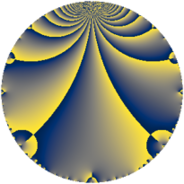# Properties

 Label 1600.2.a.cLevel $1600$ Weight $2$ Character orbit 1600.a Self dual yes Analytic conductor $12.776$ Analytic rank $1$ Dimension $1$ CM no Inner twists $1$

# Related objects

## Newspace parameters

 Level: $$N$$ $$=$$ $$1600 = 2^{6} \cdot 5^{2}$$ Weight: $$k$$ $$=$$ $$2$$ Character orbit: $$[\chi]$$ $$=$$ 1600.a (trivial)

## Newform invariants

 Self dual: yes Analytic conductor: $$12.7760643234$$ Analytic rank: $$1$$ Dimension: $$1$$ Coefficient field: $$\mathbb{Q}$$ Coefficient ring: $$\mathbb{Z}$$ Coefficient ring index: $$1$$ Twist minimal: no (minimal twist has level 20) Fricke sign: $$1$$ Sato-Tate group: $\mathrm{SU}(2)$

## $q$-expansion

 $$f(q)$$ $$=$$ $$q - 2q^{3} - 2q^{7} + q^{9} + O(q^{10})$$ $$q - 2q^{3} - 2q^{7} + q^{9} + 2q^{13} + 6q^{17} + 4q^{19} + 4q^{21} - 6q^{23} + 4q^{27} - 6q^{29} - 4q^{31} + 2q^{37} - 4q^{39} + 6q^{41} - 10q^{43} + 6q^{47} - 3q^{49} - 12q^{51} - 6q^{53} - 8q^{57} - 12q^{59} - 2q^{61} - 2q^{63} + 2q^{67} + 12q^{69} - 12q^{71} - 2q^{73} + 8q^{79} - 11q^{81} + 6q^{83} + 12q^{87} - 6q^{89} - 4q^{91} + 8q^{93} - 2q^{97} + O(q^{100})$$

## Embeddings

For each embedding $$\iota_m$$ of the coefficient field, the values $$\iota_m(a_n)$$ are shown below.

For more information on an embedded modular form you can click on its label.

Label $$\iota_m(\nu)$$ $$a_{2}$$ $$a_{3}$$ $$a_{4}$$ $$a_{5}$$ $$a_{6}$$ $$a_{7}$$ $$a_{8}$$ $$a_{9}$$ $$a_{10}$$
1.1
 0
0 −2.00000 0 0 0 −2.00000 0 1.00000 0
 $$n$$: e.g. 2-40 or 990-1000 Significant digits: Format: Complex embeddings Normalized embeddings Satake parameters Satake angles

## Atkin-Lehner signs

$$p$$ Sign
$$2$$ $$1$$
$$5$$ $$1$$

## Inner twists

This newform does not admit any (nontrivial) inner twists.

## Twists

By twisting character orbit
Char Parity Ord Mult Type Twist Min Dim
1.a even 1 1 trivial 1600.2.a.c 1
4.b odd 2 1 1600.2.a.w 1
5.b even 2 1 320.2.a.f 1
5.c odd 4 2 1600.2.c.d 2
8.b even 2 1 100.2.a.a 1
8.d odd 2 1 400.2.a.c 1
15.d odd 2 1 2880.2.a.m 1
20.d odd 2 1 320.2.a.a 1
20.e even 4 2 1600.2.c.e 2
24.f even 2 1 3600.2.a.be 1
24.h odd 2 1 900.2.a.b 1
40.e odd 2 1 80.2.a.b 1
40.f even 2 1 20.2.a.a 1
40.i odd 4 2 100.2.c.a 2
40.k even 4 2 400.2.c.b 2
56.h odd 2 1 4900.2.a.e 1
60.h even 2 1 2880.2.a.f 1
80.k odd 4 2 1280.2.d.g 2
80.q even 4 2 1280.2.d.c 2
120.i odd 2 1 180.2.a.a 1
120.m even 2 1 720.2.a.h 1
120.q odd 4 2 3600.2.f.j 2
120.w even 4 2 900.2.d.c 2
280.c odd 2 1 980.2.a.h 1
280.n even 2 1 3920.2.a.h 1
280.s even 4 2 4900.2.e.f 2
280.bf even 6 2 980.2.i.i 2
280.bk odd 6 2 980.2.i.c 2
360.bh odd 6 2 1620.2.i.b 2
360.bk even 6 2 1620.2.i.h 2
440.c even 2 1 9680.2.a.ba 1
440.o odd 2 1 2420.2.a.a 1
520.p even 2 1 3380.2.a.c 1
520.bo odd 4 2 3380.2.f.b 2
680.h even 2 1 5780.2.a.f 1
680.be even 4 2 5780.2.c.a 2
760.b odd 2 1 7220.2.a.f 1
840.u even 2 1 8820.2.a.g 1

By twisted newform orbit
Twist Min Dim Char Parity Ord Mult Type
20.2.a.a 1 40.f even 2 1
80.2.a.b 1 40.e odd 2 1
100.2.a.a 1 8.b even 2 1
100.2.c.a 2 40.i odd 4 2
180.2.a.a 1 120.i odd 2 1
320.2.a.a 1 20.d odd 2 1
320.2.a.f 1 5.b even 2 1
400.2.a.c 1 8.d odd 2 1
400.2.c.b 2 40.k even 4 2
720.2.a.h 1 120.m even 2 1
900.2.a.b 1 24.h odd 2 1
900.2.d.c 2 120.w even 4 2
980.2.a.h 1 280.c odd 2 1
980.2.i.c 2 280.bk odd 6 2
980.2.i.i 2 280.bf even 6 2
1280.2.d.c 2 80.q even 4 2
1280.2.d.g 2 80.k odd 4 2
1600.2.a.c 1 1.a even 1 1 trivial
1600.2.a.w 1 4.b odd 2 1
1600.2.c.d 2 5.c odd 4 2
1600.2.c.e 2 20.e even 4 2
1620.2.i.b 2 360.bh odd 6 2
1620.2.i.h 2 360.bk even 6 2
2420.2.a.a 1 440.o odd 2 1
2880.2.a.f 1 60.h even 2 1
2880.2.a.m 1 15.d odd 2 1
3380.2.a.c 1 520.p even 2 1
3380.2.f.b 2 520.bo odd 4 2
3600.2.a.be 1 24.f even 2 1
3600.2.f.j 2 120.q odd 4 2
3920.2.a.h 1 280.n even 2 1
4900.2.a.e 1 56.h odd 2 1
4900.2.e.f 2 280.s even 4 2
5780.2.a.f 1 680.h even 2 1
5780.2.c.a 2 680.be even 4 2
7220.2.a.f 1 760.b odd 2 1
8820.2.a.g 1 840.u even 2 1
9680.2.a.ba 1 440.c even 2 1

## Hecke kernels

This newform subspace can be constructed as the intersection of the kernels of the following linear operators acting on $$S_{2}^{\mathrm{new}}(\Gamma_0(1600))$$:

 $$T_{3} + 2$$ $$T_{7} + 2$$ $$T_{11}$$ $$T_{13} - 2$$

## Hecke characteristic polynomials

$p$ $F_p(T)$
$2$ $$T$$
$3$ $$2 + T$$
$5$ $$T$$
$7$ $$2 + T$$
$11$ $$T$$
$13$ $$-2 + T$$
$17$ $$-6 + T$$
$19$ $$-4 + T$$
$23$ $$6 + T$$
$29$ $$6 + T$$
$31$ $$4 + T$$
$37$ $$-2 + T$$
$41$ $$-6 + T$$
$43$ $$10 + T$$
$47$ $$-6 + T$$
$53$ $$6 + T$$
$59$ $$12 + T$$
$61$ $$2 + T$$
$67$ $$-2 + T$$
$71$ $$12 + T$$
$73$ $$2 + T$$
$79$ $$-8 + T$$
$83$ $$-6 + T$$
$89$ $$6 + T$$
$97$ $$2 + T$$